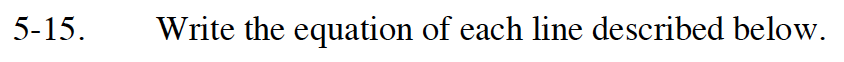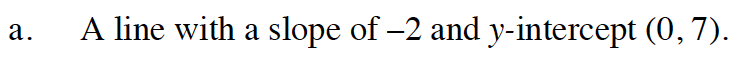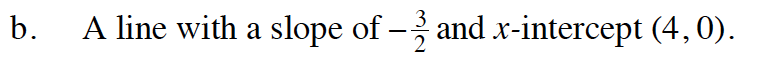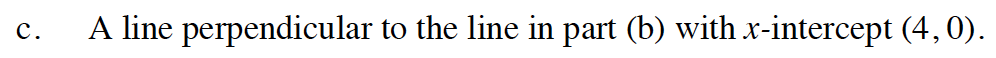Home > INT1 > Chapter Ch5 > Lesson 5.1.1 > Problem5-15

5-15.
1. Write the equation of each line described below. Homework Help ✎

1. A line with a slope of –2 and y-intercept (0, 7).

2. A line with a slope of –and x-intercept (4, 0).

3. A line perpendicular to the line in part (b) with x-intercept (4, 0).Remember that Slope-Intercept form is y = mx + b.$\text{Substitute }-\frac{3}{2}\text{ for }m\text{ in }y=mx+b$

Substitute 4 for x and 0 for y.

0 = −6 + b

Solve for b.

b = 6

$\textit{y}=-\frac{3}{2}\textit{x}+6$